Most Affordable JEE | NEET | 8,9,10 Preparation by Kota's Top IITian Doctor Faculties

# d & f -block Elements | Question Bank for Class 12 Chemistry`
Q. Silver atom has completely filled $d$ -orbitals $\left(4 d^{10}\right)$ in its ground state. How can you say that it is a transition element.
Ans. The outer electronic configuration of $A g(Z=47)$ is $4 d^{10} 5 s^{1} .$ In addition to $+1,$ it shows an oxidation state of $+2$ (e.g., $A g O$ and $A g F_{2}$ exist). In $+2$ oxidation state, the configuration is $d^{9},$ i.e. the $d$ -subshell is incompletley filled. Hence, it is a transition element.
Q. Why is the highest oxidation state of a metal exhibited in its oxide or fluoride only?
Ans. Oxygen and fluorine have small size and high electronegativity. Hence, they can oxidize the metal to the highest oxidation state.
Q. Which is a stronger reducing agent $C u^{2+}$ or $F e^{2+}$ and why?
Ans. $C r^{2+}$ is a stronger reducing agent than $F e^{2+}$ Reason: $E_{C r}^{\circ} / C r^{2+}$ is -ve $(-0.41 \mathrm{V})$ whereas $E_{F e^{3+} / F e^{2+}}^{\circ}$ is $+v e$ $(+0.77 V) .$ Thus, is easily oxidized to but cannot be easily oxidized to. Hence, is stronger reducing agent than.
Q. Calculate the 'spin only' magnetic moment of $M_{(a q)}^{2+}$ ion $(Z=27)$
Ans. Electronic configuration of $M$ atom with $Z=27$ is $[A r] 3 d^{7} 4 s^{2}$ $\therefore$ Electronic configuration of $M^{2+}$ will be $[A \eta] 3 d^{7},$ i.e.,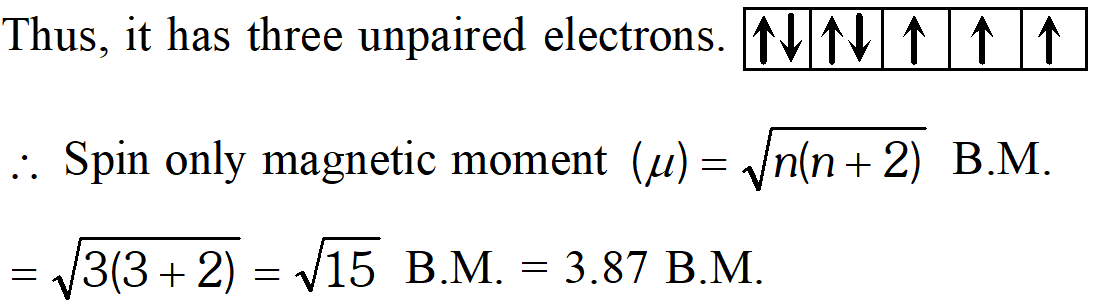Q. Actinoid contraction is greater from element to elements than lanthanoid contraction. Why?
Ans. This is due to poor shielding by $5 f$ electrons in the actionids than that by $4 f$ electrons in the lanthanoids.
Q. Why do the transition elements exhibit higher enthalpies of atomisation?
Ans. Because of large number of unpaired electrons in their atoms they have stronger interatomic interaction and hence stronger bonding between atoms resulting in higher enthalpies of atomisation.
Q. Name a transition element which does not exhibit ‘variable’ oxidation states.
Ans. Scandium (Z = 21) does not exhibit variable oxidation states.
Q. How would you account for the increasing oxidising power in the series $V O_{2}^{+} Ans. This is due to the increasing stability of the lower species to which they are reduced. Q. Why is the$E^{y}$value for the$M n^{3+} / M n^{2+}$couple much more positive than that for$C r^{3+} / C r^{2+}$or$F e^{3+} / F e^{2+7} ?$Explain. Ans. Much larger ionisation energy of$M n$(where the required change is to$d^{4}$) is mainly responsible for this. This also explains why$d^{5}$the$+3$state of$M n$is of little importance. Q. Calculate the magnetic moment of a divalent ion in aqueous solution if its atomic number is 25 Ans. With atomic number$25,$the divalent ion in aqueous solution will have$d^{5}$configuration (five unpaired electrons). The magnetic moment,$\mu$is$\mu=\sqrt{5(5+2)}=5.92 B M$Q. Name a member of the lanthanoid series which is well known to exhibit$+4$oxidation state. Ans. Cerium$(Z=58)$Q. What may be the stable oxidation state of the transition element with the following$d$-electron configurations in the ground state of their atoms :$3 d^{\beta}, 3 d^{8}, 3 d^{8}, 3 d^{4} ?$Ans. - For,$3 d^{3} 4 s^{2},$the most stable oxidation state is$+5$For,$3 d^{5} 4 s^{2},$the stable oxidation states are$+2$and$+7$For,$3 d^{8} 4 s^{2},$the stable oxidation state is$+2$For,$3 d^{4} 4 s^{2},$the stable oxidation states are$+3$and$+6$Q. Name of the oxometal anions of the first series of the transition metals in which the metal exhibits the oxidation state equal to its group number. Ans. Chromate ion,$C r O_{4}^{2-}$- Oxidation state$+\mathrm{VI}$Dichromate ion,$C r_{2} O_{7}^{2-}-$Oxidation state$+\mathrm{VI}$Permanganate ion,$M n O_{4}^{-}$- Oxidation state$+\mathrm{VII}$Group number of chromium is 6 while that of manganese is 7 . Q. What are the different oxidation states exhibited by the lanthanoids ? Ans. The principal oxidation state of lanthanoids is$+3 .$However, some lanthanoids also exhibit oxidation state of$+2$and$+4 .$For example, Eu exhibit oxidation state of$+2$and$C e$exhibits oxidation state of$+4$Q. Which metal in the first series of transition metals exhibits$+1$oxidation state most frequently and why? Ans. In the first transition series copper exhibit$+1$oxidation state most frequently. This is due to the reason that$C u($I) has stable electronic configuration.$C u(I):[A r] 3 d^{10}$Q. In the series$S c(Z=21)$to$\operatorname{Zn}(Z=30),$the enthalpy of atomisation of zinc is the lowest, i.e.,$126 \mathrm{kJ} \mathrm{mol}^{-1} .$Why? Ans. - In the series,$S c$to$Z n,$all elements have one or more unpaired electrons except zinc which has no unpaired electron as its outer electronic configuration is$3 d^{10} 4 s^{2} .$Hence, atomic intermetallic bonding (metal-metal bonding) is weakest in zinc. Therefore, enthalpy of atomisation is lowest. Q. Which of the$3 d$series of the transition metals exhibits the largest number of oxidation states and why? Ans. Manganese$(Z=25)$shows maximum number of oxidation states. This is because its electronic configuration is$3 d^{5} 4 s^{2} .$As$3 d$and 4s are close in energy, it has maximum number of electrons to lose or share (as all the electrons are unpaired). Hence, if shows oxidation states from$+2$to$+7(+2,+3,+4,+5,+6 \text { and }+7)$which is the$\mathrm{n}$um number. Q. How would you account for the irregular variation of ionization enthalpies (first and second) in first series of the transition elements? Ans. Irregular variation of first ionization enthalpy : As we move from left to right along the first transition series, as effective nuclear change increases, it is expected in general that the first ionization enthalpy should show an increasing trend. However, the trend is irregular because removal of the electron alters the relative energies of$4 s$and$3 d$orbitals. Thus, there is a reorganisation energy accompanying ionization. This results into the release of exchange energy which increase as the number of electrons increases in the$d^{n}$configuration and also from the transference of$s$-electrons into$d$-orbitals.$C r$has low first ionization energy because loss of one electron gives stable electronic configuration$\left(3 d^{5}\right) . Z n$has very high ionization energy because electron has to be removed from orbital of the stable configuration. Irregularities of second ionization enthalpy : After the loss of one electron, the removal of second electron becomes difficult. Hence, second ionization enthalpies are much higher and in general increase from left to right. However, and show much higher values because the second electron has to be removed from the stable configurations of and. Q. The$E^{o}\left(M^{+} / M\right)$value for copper is positive$(+0.34 \mathrm{V}) .$What is possibly the reason for this? Ans.$E^{o}\left(M^{2+} / M\right)$for any metal is related to the sum of the enthalpy changes taking place in the following steps:$M(s)+\Delta_{a} H \rightarrow M(g),( \left\Delta_{a} H=$enthalpy of atomisation) \right.$M(g)+\Delta_{i} H \rightarrow M^{2+}(g),\left(\Delta_{i} H=\text { ionization enthalpy }\right)M^{2+}(g)+a q \rightarrow M^{2+}(a q)+\Delta_{h y d} H( \left\Delta_{h y d} H=$hydration \right. enthalpy$)$Copper has high enthalpy of atomisation (i.e., energy absorbed) and low enthalpy o hydration, (i.e., energy released). Hence,$E^{o}\left(C u^{2+} / C u\right)$is positive. The high energy required to transform$C u(s)$to$C u^{2+}(a q)$is not balanced by its hydration enthalpy. Q. Explain why$C u^{+}$ion is not stable in aqueous solution? Ans.$\mathrm{Cu}^{2+}(a q)$is much more stable than$\mathrm{Cu}^{+}(a q) .$This is because although second ionization enthalpy of copper is large but$\Delta_{h y d} H$for$C u^{2+}(a q)$is much more negtive than that for and hence it is more than compensates for the second ionization enthalpy of copper. Therefore, many copper (I) compounds are unstable in aqueous solution and undergo disproportionation as follows:$2 \mathrm{Cu}^{+} \longrightarrow \mathrm{Cu}^{2+}+\mathrm{Cu}$Q. On what ground can you say that scandium (Z= 21) is a transition element but zinc (Z = 30) is not ? Ans. On the basis of incompletely filled$3 d$orbitals in case of scandium atom in its ground state$\left(3 d^{1}\right),$it is regarded as a transition element. On the other hand, zinc atom has completely filled$d$orbitals$\left(3 d^{10}\right)$in its ground state as well as in its oxidised state, hence it is not regarded as a transition element. Q. Why is$C r^{2+}$reducing and$M n^{3+}$oxidising when both have$d^{4}$configuration? Ans.$C r^{2+}$is reducing as its configuration changes from$d^{4}$to$d^{3},$the latter having half-filled$t_{2 g}$level. On the other hand, the change from$M n^{2+}$to$M n^{3+}$results in the half-filled$\left(d^{5}\right)$configuration which has extra stability. Q. For the first row transition metals the$E^{\circ}$values are: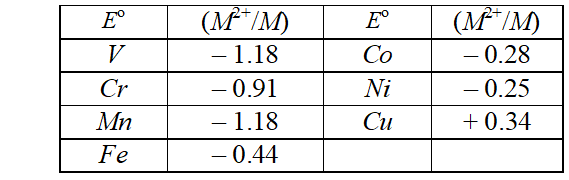Explain the irregularity in the above values. Ans. The$E^{\ominus}\left(M^{2+} / M\right)$values are not regular which can be explained from the irregular variation of ionisation enthalpies$\left(\Delta_{i} H_{1}+\Delta_{i} H_{2}\right)$and also the sublimation enthalpies which are relatively much less for manganese and vanadium. Q. What is meant by ‘disproportionation’ of an oxidation state? Give an example. Ans. When a particular oxidation state becomes less stable relative to cother oxidation states, one lower, one higher, it is said to undergo disproportionation. For example, manganese (VI) becomes unstable Telative to manganese (VII) and manganese (IV) in acidic solution.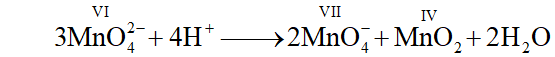Q. Write down the electronic configuration of the following ions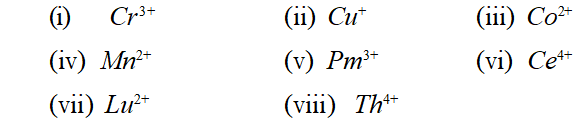(Atomicnos. are:$C r=24, C u=29, C o=27, M n=25, P m=61C e=58, L u=71, T h=90)$Ans.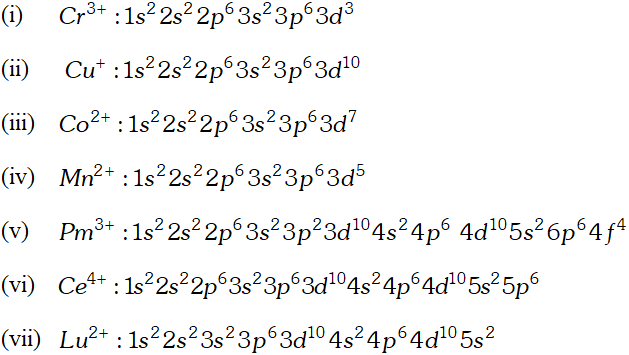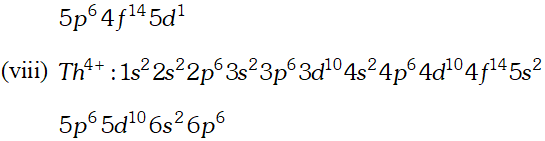Q. Why are$M n^{2+}$compound more stable than$F e^{2+}$towards oxidation to their$+3$state? Ans. The electronic configuration of$M n^{2+}$is$3 d^{5},$which is stable due to half filled sub shell. Hence, is not easily oxidized to. On the other hand, has electronic configuration$3 \mathrm{d}^{6} .$After losing one electron it change to$\mathrm{Fe}^{3+}$which has stable electronic confi-guretion. Hence,$\mathrm{Fe}^{2+}$is relatively easily oxidized to$\mathrm{Fe}^{3+}$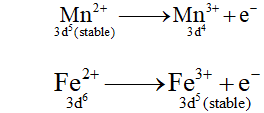Q. Explain briefly how$+2$state becomes more and more stable in the first half of the first row transition elements with increasing atomic number? Ans. Except scandium, the most common oxidation state of the first row transition elements is$+2$which arises from the loss of two$4 s$electrons. The$+2$state becomes more and more stable in the first half of first transition elements with increasing atomic number because$3 d$orbitals acquire only one electron in each of five$3 d$orbitals (i.e., each$d$-orbital remains half filled) and electronic repulsion is the least and nuclear charge increases. In second half of first row transition elements, electronstarts pairing up in 3$d$orbitals.$\left(T i^{2+} \text { to } M n^{2+} \text { electronic configuration changes from } 3 d^{2} \text { to } 3 d^{5}\right.$but in second half i.e.,$F e^{2+}$to$Z n^{2+}$it changes from$3 d^{6}$to$\left.3 d^{10}\right)$Q. To what extent do the electronic configurations decide the stability of oxidation states in the first series of the transition elements? Illustrate your answer with example. Ans. The stability of an oxidation state is determined to a large extent by the electronic configuration of the element, in case of transition elements. Those oxidation states in which the element acquires estable configuration such as noble gas configuration, exactly halffilled or fully filled$d$-subshell, are exceptionally stable. For example,$S c^{3+}, M n^{2+}$and$Z n^{2+}$are relatively more stable.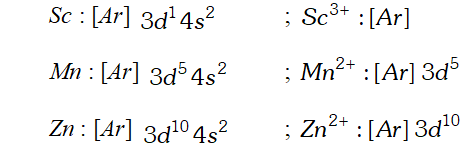Q. What is lanthanoid contraction? What are the consequences of lanthanoid contraction? Ans. The atomic radii and ionic radii of tripositive lanthanoidions$\left(M^{3+}\right)$show a steady and gradual decrease in moving from La to Lu. Although the atomic radii do show some irregularities but ionic radii decreases steadily from$L a$to$L u .$The steady decrease in size of lanthanoid ions with the increase in atomic number is called lanthanoid contraction. Consequences of Lanthanoid Contraction : (i) Similarity of second and third transition series : The atomic radii of second row of transition elements are almost similar to those of the third row of transition elements. (ii) Variation in basic strength of hydronides : The basic strength of hydroxides decreases from to . Due to lanthanoid contraction size of ions decreases and there is increase in the covalent character in MOH bond. (iii) Separation of lanthanoids : Separation of lanthanoids is also possible due to lanthanoid contraction. All the lanthanoid have quite similar properties and due to this reason they are difficult to be separated. However, because of lanthanoid contraction their properties vary slightly. Q. In what way is the electronic configuration of the transition elements different from that of the non-transition elements? Ans. Transition elements have partially filled d-subshell belonging to penultimate energy level whereas non-transition elements do not have any partially filled d-subshell. In non-transition elements, the last electron enters the s or p-subshell whereas in transition elements the last electron enters the d-subshell of penultimate energy level. Q. Explain giving reason : (i) Transition metals and many of their compounds show paramagnetic behaviour. (ii) The enthalpies of ionisation of the transition metal are high. (iii) The transition metals generally form coloured compounds. (iv) Transition metals and their many compounds act as good catalyst. Ans. (i) The transition metal ion generally contain one or more unpaired electrons in them and hence their complexes are generally paramagnetic. (ii) The ionization enthalpies of transition elements are higher than those of s-block elements but lower than p-block elements. In a particular transition series, ionization enthalpy increases gradually as we move from left to right. The increase in ionization enthalpy is particularly due to increase in nuclear charge. (iii) The d-orbitals in the transition elements do not have same energy in their complexes under the influence of the ligands attached, the d-orbitals split into two sets of orbitals having slightly different energies ‘In the transition elements which have partly filled’. d-orbitals, the transition of elements can take place from one of the lower d-orbitals to some higher d-orbital within the same sub-shell. The energy required for this transition falls in the visible region. When light falls on these complexes they absorb a particular colour from the radiation for the promotion of electron and the remaining colours are emitted. (iv) The catalyst activity of transition metals is attributed to the following reasons (a) Because of their variable valencies transition metals sometimes form unstable intermediate compounds and provide a new path with lower activation energy for the reaction. (b) In some cases transition metals provide a suitable surface of the reaction to take place. The reactants are adsorbed on the surface of the catalyst where reactions occurs. Q. What are interstitial compounds? Why are such compounds well known for transition metals ? Ans. Interstitial compounds are those which are formed when small atoms like H, C, N, B etc. are trapped inside the crystal lattices of metals. They are generally non-stoichiometric. Most of transition metals form interstitial compounds with small non-metal atoms such as hydrogen, boron, carbon and nitrogen. These small atoms enter into the interstitial voids between the lattice of transition metals and from chemical bonds with transition metals. For examples, steel and cast iron becomes hard by forming interstitial compound with carbon. The existence of vacant d-orbitals in transition elements and their ability to make bonds with trapped small atoms is the main cause of interstitial compound formation. Q. How is the variability in oxidation states of transition metal different from that of the non-transition metals? illustration with examples. Ans. In case of transition elements, the oxidation states of differ from each other by unity whereas in case of non-transition elements oxidation states normally differ by units of two. For example, Fe exhibits oxidation states of$+2$and$+3 .$Similarly,$C u$exhibits oxidations states of$+1$and$+2 .$On the other hand,$S n$and$P b$exhibit oxidation states of$+2$and$+4$Q. Describe the preparation of$K_{2} C r_{2} O_{7}$from iron chromite ore. What is the effect of increasing$p H$on a solution of. Ans. Step I : Preparation of sodium chromate$4 \mathrm{FeCr}_{2} \mathrm{O}_{4}+16 \mathrm{NaOH}+7 \mathrm{O}_{2} \longrightarrow8 \mathrm{Na}_{2} \mathrm{CrO}_{4}+2 \mathrm{Fe}_{2} \mathrm{O}_{3}+8 \mathrm{H}_{2} \mathrm{O}$Step II : Conversion of sodium chromate into sodium dichromate$2 \mathrm{Na}_{2} \mathrm{CrO}_{4}+\mathrm{H}_{2} \mathrm{SO}_{4} \longrightarrow \mathrm{Na}_{2} \mathrm{Cr}_{2} \mathrm{O}_{7}+\mathrm{Na}_{2} \mathrm{SO}_{4}+\mathrm{H}_{2} \mathrm{O}$Step III : Conversion of sodium dichromate into potassium dichromate$\mathrm{Na}_{2} \mathrm{Cr}_{2} \mathrm{O}_{7}+2 \mathrm{KCl} \longrightarrow \mathrm{K}_{2} \mathrm{Cr}_{2} \mathrm{O}_{7}+2 \mathrm{NaCl}$Effect of increasing$p H$on a solution of$\boldsymbol{K}_{2} \mathbf{C r}_{2} \mathbf{O}_{7}$On increasing$p H$of solution dichromate ions, change into chromate ion.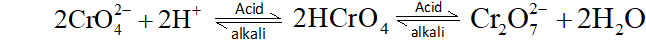Q. Describe the oxidising action of potassium dichromate and write the ionic equation for its reaction with (i) Iodide (ii) Iron (II) solution$\quad$(iii)$H_{2} S$Ans. Potassium dichromates,$K_{2} C r_{2} O_{7}$is a strong oxidising agent. In acidic solution, its oxidising action can be represented as follows: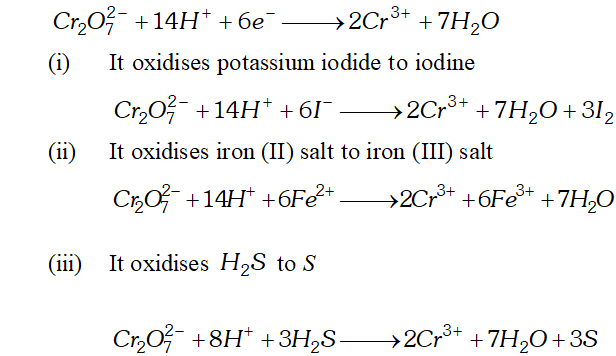Q. Describe the preparation of potassium permangnate. How does the acidified permanganate solution reacts with (i) iron (II) ion (ii) Oxalic acid? Write the ionic equations for reactions. Ans.$-K M n O_{4}$is prepared from pyrolusite$\left(M n O_{2}\right)$Pyrolusite is fused with$K O H$in the presence of oxygen or an oxidizing agent like potassium nitrate or potassium chlorate to give potassium manganate,$K_{2} M n O_{4}$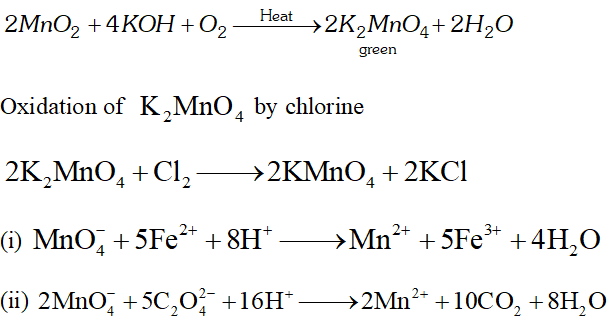Q. For$M^{2+} / M$and$M^{3+} / M^{2+}$systems the$E^{\mathrm{E}}$values for some metals are as follows: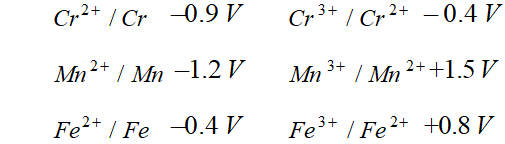Use this data to comment upon: (i) The stability of$F e^{3+}$in acid solution as compared to that of$C r^{3+}$or$M n^{3+}$(ii) The ease with which iron can be oxidised as compared to the similar process for either chromium or manganese metal. Ans. (i) We know that higher the reduction potential of species, larger is the tendency for its reduction to take place. Thus,$M n^{3+}$with highest reduction potential would be most readily reduced to$M n^{2+}$and hence is least stable. From values of reduction potential, it is clear that the stability of$F e^{3+}$in acid solution is more than that but less than that of. (ii) The lower the reduction potential of a species, greater is the ease with which its oxidation will take place.$\therefore M n$is oxidised most readily to and$F e$is oxidised to least readily among given metals$M n, C r$and$F e .$Thus, order of their tendency to undergo oxidation is$\mathrm{Mn}>\mathrm{Cr}>\mathrm{Fe}$Q. Predict which of the following will be coloured in aqueous solution?$T i^{3+}, V^{3+}, C u^{+}, S c^{3+}, M n^{2+}, F e^{3+}, C o^{2+}$and$M n O_{4}^{-}$Give reason for each. Ans. - Any ion that has partially filled$d$-orbitals is coloured due to$d-d$transition in visible light.$T i^{3+}(d), V^{3+}\left(d^{2}\right), M n^{2+}\left(d^{5}\right)F e^{3+}\left(d^{6}\right), C o^{2+}\left(d^{8}\right)$are coloured.$M n O_{4}^{-}$has purple colour due to change transfer.$C u^{+}\left(d^{10}\right)$and$S c^{3+}\left(d^{0}\right)$are white.b Q. Compare the stability of$+2$oxidation state for the elements of the first transition series. Ans. In the first transition series the stability of$+2$oxidation state of elements, in general, decreases from left to right. This is due to increase in the value of sum of$I E_{1}$and$I E_{2} .$However,$M n$and$Z n$show exceptional behaviour. The greater stability of$+2$oxidation states of$M n$and$Z n$are due to half filled$d$-subshell$\left(d^{5}\right)$in$M n^{2+}$and fully filled$d$-subshell$\left(d^{10}\right)$in$Z n^{2+}$Q. How would you account for the following: (1) Of the$d^{4}$species, chromium (II) is strongly reducing while manganese (III) is strongly oxidising. (ii) Cobalt (II) is stable in aqueous solution but in the presence of complexing regents it is easily oxidised. (iii) The$d^{1}$configuration is very unstable in ions. Ans. (i) For chromium,$+$III state is more stable as compared to$+\Pi$state. Therefore,$C r^{2+}$readily change into$C r^{3+}$and behaves as a strong reducing agent. On the other hand, for manganese, + II state is more stable than$+$III state. Hence,$M n^{3+}$readily changes into$M n^{2+}\left(3 d^{5}\right)$by gaining an electron and behave as an strong oxidising agent. (ii) In the presence of ligands$\mathrm{Co}(\mathrm{II})$is oxidised to$\mathrm{Co}(\mathrm{III})$which has$d^{6}$configuration. Most of the ligands are strong enough to cause spin paring giving rise to diamagnetic octahedral complexes which are very stable and have very large crystal field stabilization energy. (iii) The ions in$d^{1}$configuration have great tendency to acquire more stable$d^{0}$configuration by losing the lone$d$-electron and act as reducing agent. In some cases, the ion in configuration undergoes disproportionation. For example,$3 \mathrm{MnO}_{4}^{2-}+4 \mathrm{H}^{+} \longrightarrow 2 \mathrm{MnO}_{4}^{-}+\mathrm{MnO}_{2}+2 \mathrm{H}_{2} \mathrm{O}$Q. What is meant by 'disproportionation'? Give two examples of disproportiantion reaction in aqueous solution. Ans. Sometimes a particular oxidation state becomes less stable relative to other oxidation states, one lower and the other higher. In such a situation a part of the species undergoes oxidation while a part undergoes reduction. Such a species is said to undergo disproportionation.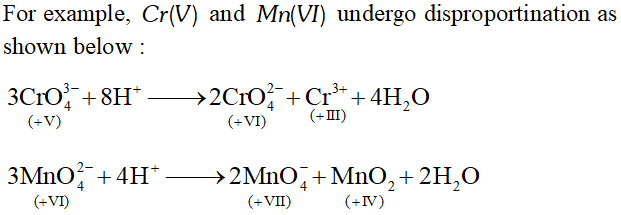Thus,$C r(V)$undergoes disproportionation to$C r(V I)$and$C r(\mathrm{III})$while$M n(\mathrm{VI})$undergoes disproportionation to$M n(\mathrm{VII})$and$M n(\mathrm{IV})$Q. Calculate the number of unpaired electrons in following gaseous ions :$M n^{3+}, C r^{3+}, V^{3+},$and$T i^{3+} .$Which one of these is the most stable in aqueous solution? Ans.$M n^{3+}:[A r] 3 d^{4} ;$four unpaired electrons$C r^{3+}:[A r] 3 d^{3} ;$three unpaired electrons$V^{3+}:[A r] 3 d^{2} ;$two unpaired electrons$T i^{3+}:[A r] 3 d^{1} ;$one unpaired electron. Out of these$C r^{3+}$is most stable in aqueous solution. Q. Give example and suggest reasons for the following features of the transition metal chemistry : (i) The lowest oxide of transition metal is basic, the highest is acidic. (ii) A transition metal exhibits higher oxidation states in oxides and fluorides. (iii) The highest oxidation state is exhibited in oxoanions of a metal. Ans. (i) Transition metal oxides in lowest oxidation state are basic and in higher oxidation states become acidic in nature. This is illustrated by following example of various oxides of manganese: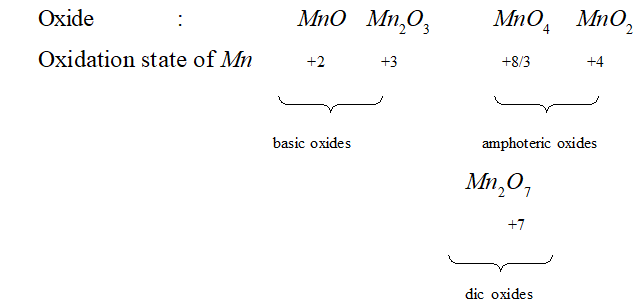In low oxidation state of metal some of its electrons are not involved in bonding and hence its effective nuclear charge is not very high and its oxide can denote electrons and behave as a base. On the other hand when the metal is in higher oxidation state, its effective nuclear charge is very high and hence its oxide has tendency to gain electrons and behaves as an acid. (ii) It is due to the fact that fluorine and oxygen are the two most electronegative elements. (iii) The highest oxidation state of transition element is seen in oxides and these oxides. Dissolve in acids and bases and form oxoanions of transition metal. Thus oxoanions of transition element show highest oxidation state of transition metal. For example, the highest oxidation state of$M n$is$+7$and it is observed in its oxoanion$M n O_{4}^{-}$(permanganate ion). The highest oxidation state of$C r$is$+6$and it is observed in its oxoanions,$C r O_{4}^{2-}$(chromate ion) and$C r_{2} O_{7}^{2-}$(dichromate ion). Q. What are alloys? Name an important alloy which contains some of the lanthanoid metals. Mention its uses. Ans. An alloy is a homogeneous mixture of a metal with other metals or non-metals. A large number of alloys of transition metals are known and extensively used in modern industries. An important alloy which contains some of lanthanoid metals is "misch-metal" Uses of Misch-Metal: (i) Addition of about$3 \%$misch metal to magnesium increases its strength and thus is used in making jet engine parts. (ii) It is pyrophoric and is used in cigarette and gas lighters, tracer bullets, shells, etc. Q. What are inner transition elements? Describe which of the following atomic numbers are the atomic numbers of the inner transition elements:$29,59,74,95,102,104$Ans. - Inner transition elements are the elements which have partly filled$f$-orbitals. These are also called$f$-block elements. There are two series of inner transition elements. (i) Lanthanoids ($4 f$series) : These are the 14 elements from atomic number$58-71$(ii) Actinoids: ($5 f$series ): These are the 14 elements from atomic number$90-103$Among the given atomic numbers, only 5995 and 102 are the atomic number of inner transition elements. Q. The chemistry of the actinoid element is not so smooth as that of the lanthanoids. Justify this statement by giving some example from the oxidation state of these elements. Ans. - Among the actinoids, there is a greater range of oxidation states as compared to lanthanoids. This is in part due to the fact that$5 f, 6 d$and$7 s$sub-shells are of comparable energies and the frequent electronic transition among these three sub-shells is possible. The$6 d-5 f$transition and large number of oxidation states among actinoids make their chemistry more complicated. All actinoids in general exhibit$+3$oxidation state. The elements in the first half of the series frequently exhibit higher oxidation state. The maximum oxidation state increases from$+4$in$T h$to$+5,+6$and$+7$respectively in$P a, U$and$N p$but decreases in the succeeding elements. since the distribution of oxidation states among the actionoids is so uneven and so different for the earlier and later elements, as a result their chemistry is not so smooth as that of lanthanoids. Q. Which is the last element in the series of the actinoids? Write the electronic configuration of this element. Comment on the possible oxidation state of this element. Ans. - Lawrencium (Lr) is the last element in the series of the actinoids. Its electronic configuration is$L r:[R n] 5 f^{14} 6 d^{1} 7 s^{2}$The possible oxidation state of$L r$is$+3$which has stable electronic configuration$[R n] 5 f^{14}$Q. Use Hund's rule to derive the electronic configuration of$C e^{3+}$ion, and calculate its magnetic moment on the basis of 'spin-only' formula. Ans. - The electronic configurations of$C e$and$C e^{3+}$ion are:$C e(Z=58)=_{54}[X e] 4 f^{1} 5 d^{1} 6 s^{2}\mathrm{Ce}^{3+}=_{54}[\mathrm{Xe}] 4 \mathrm{f}^{1}$It has, one unpaired electron. 'Spin only' formula for magnetic moment of a species,$\mu=\sqrt{\mathrm{n}(\mathrm{n}+2)} \mathrm{B} \cdot \mathrm{M}$Where$n=$no. of unpaired electrons$\therefore$Magnetic moment of$\mathrm{Ce}^{3+}, \mu=\sqrt{1(1+2)}=\sqrt{3} \mathrm{B} \cdot \mathrm{M} \cdot=1.732 \mathrm{B} \cdot \mathrm{M}$Q. Name the members of the lanthanoid series which exhibit$+4$oxidation states and those which exhibit$+2$oxidation states. Try to correlate this type of behaviour with the electronic configuration of these elements. Ans.$+4$oxidation state : Cerium$(C e),$Preseodymium (Pr), Neodymium$(N d),$Terbium$(T b),$Dysprosium$(D y)+2$oxidation state: Neodymium (Nd), Samarium (Sm), Europium$(E u),$Thulium$(T m)$and Ytterbium$(Y b)+4$and$+2$oxidation states of lanthnoid elements arise mainly from the extra, stability of empty, half filled and fully filled$f$-subshell. For example$C e(I V)$is$4 f^{0}$and has noble gas configuration. Similarly$T b(\mathrm{IV})$and$E u(\mathrm{II})$have$4 f^{7}$configuration.$Y b(\mathrm{II})$has$4 f^{14}$configuration. Q. Write the electronic configurations of the elements with the atomic numbers$61,91,101$and 109 Ans. Atomic number$(61)=[X e] 4 f^{5} 6 s^{2}$Atomic number$(91)=_{86}[R n] 5 f^{2} 6 d^{1} 7 s^{2}$Atomic number$(101)=_{86}[R n] 5 f^{13} 7 s^{2}$Atomic number$(109)=_{86}[R n] 5 f^{14} 6 d^{7} 7 s^{2}$Q. Compare the general characteristics of the first series of the transition metals with those of the second and third series metals in the respective vertical columns. Give special emphasis on the following points: (i) Electronic configurations (ii) Oxidation states (iii) Ionisation enthalpies (iv) Atomic sizes Ans. (i) The elements of first transition series involve progressive filling of 3 dorbitals whereas that of second and third series involve filling of$4 d$and$5 d$subshell respectively. (ii) For the elements of first transition series$+2$and$+3$oxidation states are common and these elements form many stable complexes in these oxidation states. For the elements of second and third series higher oxidation states are more important. These elements form more stable compounds in higher oxidation states are more important. These elements form more stable compounds in higher oxidation states. For example,$\left[\mathrm{CrCl}_{6}\right]^{3-}$is very stable whereas no equivalent complexes of$M o$and$W$are known. On the contrary$\mathrm{O} \mathrm{SO}_{4}$and$\mathrm{PtF}_{6}$are quite stable and no corresponding compounds for first transition series are known. (iii) The metals of second and third transition series have higher Ionisation enthalpies than the elements of first series. The elements of second and third series form many compounds with$M-M$bond. (iv) The atomic radii of elements of second and third transition series are larger than those of the elements of first series. Because of lanthanoid contraction, the radii of third series are almost equal to those of second row. Q. Comment on the statement that elements of the first transition series possess many properties different from those of heavier transition elements. Ans. Some points of difference between properties of elements of the first transition series and those of hevier transition elements are given below: (i) For elements of first series$+2$and$+3$oxidation states are more common while for the heavier transition elements higher oxidation states are more common. (ii)$M-M$bonding is rare in the elements of first series but is quite common in heavier transition elements. (iii) The elements of first transition series do not form complexes with coordination no. 7 or 8 whereas heavier transition elements do so. (iv) The elements of first transition series form low spin or high spin complexes depending upon strength of the ligand field. On the other hand heavier transition elements form low spin complexes irrespective of the strength of the ligand field Q. What can be inferred from the magnetic moment values of the following complex species?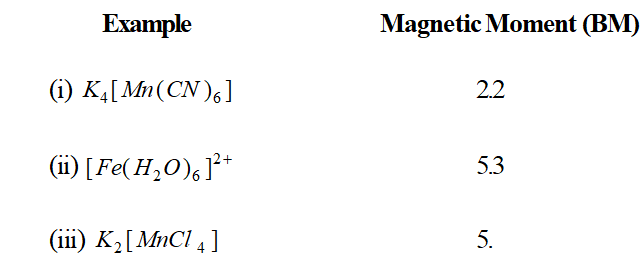Ans. Calculate the unpaired electron by applying formula$\mu=\sqrt{n(n+2)}$(i) The magnetic moment of 2.2 BM corresponds to$n=1 .$Thus, in$K_{4}\left[M n(C N)_{6}\right]$there is only one unpaired electron in$3 d$subshell. Thus, the distribution of five$3 d$electrons in$M n(\mathrm{II})$is$\left(t_{2 g}\right)^{5}$(ii) This indicates that there are four unpaired electrons in the complex. Thus, the six$3 d$electrons in$F e(\Pi)$are distributed as$\left(t_{2 g}\right)^{4}\left(e_{g}\right)^{2}$(iii) This indicates that there are five unpaired electrons in the complex. Hence, the five$3 d$electrons in$M n($II ) are distributed as$\left(t_{2 g}\right)^{3}\left(e_{g}\right)^{2}$in the given complex. Q. What are the characteristics of the transition elements and why are they called transition elements? Which of the$d-$block elements may not be regarded as the transition elements? Ans. The important characteristics of transition metals are: (i) All transition elements are metallic in nature. (ii) These metals exhibit variable oxidation states. (iii) Transition metal atoms or ions generally form the complexes with neutral, negative and positive ligands. (iv) Compounds of transition metals are usually coloured. (v) The compounds of these metals are usually paramagnetic in nature. (vi) Transition metals and their compounds act as good catalysts. (vii) These metals form various alloys with other metals of the series (viii) These metals form interstitial compounds with C, N, B and H The presence of partially filled$d$-orbitals in the electronic configuration of atomic and ionic species of these elements is responsible for the characteristic properties of transition elements. They are called transition elements because of their position in the periodic table. On one side of transition metals are highly reactive metals of s-block whereas on the side are less reactive metals of$p-$block. A transition element may be defined as a element whose atom or at least one of its simple ions contains partially filled$d-$orbitals, e.g., iron, copper, chromium, nickel etc. The elements zinc, cadmium and mercury are generally not regarded as transition elements as their atoms and all ions formed do not have partially filled$d$-orbitals in atomic state or common oxidation state$\left(Z n^{2+}, C d^{+}, H g^{2+}\right)$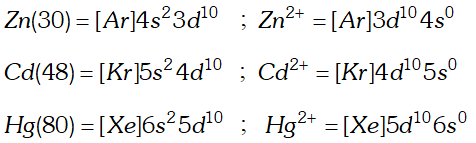Q. Compare the chemistry of actinoids with that of the lanthanoids with special reference to (i) Electronic configuration (ii) Oxidation state (iii) Atomic and ionic sizes (iv) Chemical reactivity Ans. (i) Electronic configuration: All the lanthanoids have electronic configuration with$6 s^{2}$common with variable occupancy of$4 f$and$5 d$sub-shells. All actinoids have the electronic configuration of$7 s^{2}$and variable occupancy of$5 f$and$6 d$sub-shell. (ii) Oxidation state : Lanthanoids exhibit a principal oxidation state of$+3 .$Cerium and terbium also exhibit oxidation state of$+4 . \mathrm{Sm}^{2+}, \mathrm{Eu}^{2+}$and$\mathrm{Yb}^{2+}$ions also exist in aqueous solutions. The common oxidation state of these elements is$+3 .$However, they also exhibit oxidation state of$+2,+4,+5+6$and$+7 .$Thus, actinoids exhibit greater range of oxidation states. (iii) Atomic and ionic sizes : The atomic radii and ionic radii of tripositive lanthanoid ions$\left(M^{3+}\right)$show a steady and gradual decrease in moving from La to Lu this is due to poor shielding of$4 f$-electrons. In actinoid, the steady decrease in ionic radii with increase in atomic number referred to as actinoid contraction. The contraction is greater from element to element in this series. This is due to poor shielding by$5 f$-electrons (iv) Chemical reactivity : The earlier member of the series are quite reactive similar to calcium, however with increase in atomic number their reactivity decreases and they behave more like aluminium. Many lanthanoids react with carbon to form salt like carbides and with hydrogen to give salt-like hydrides. Actinoids tarnish in air due to formation of oxide coating. They react with not water. The actinoids react with most non-metals at moderate temperatures. Q. Indicate the steps in the preparation of: (i)$\quad K_{2} C r_{2} O_{7}$from chromite ore (ii)$K M n O_{4}$from pyrolusite ore. Ans. (i)$\quad \mathrm{K}_{2} \mathrm{Cr}_{2} \mathrm{O}_{7}$from chromite ore: Following various steps are involved in the preparation of from chromite ore. (a) Preparation of sodium chromate: The powdered ore is heated with molten alkali in the presence of air in a reverberatory furnance to produce sodium chromate.(b) Conversion of sodium chromate in to sodium dichromate: Sodium chromate is extracted with water and acidified with sulphuric acid to get sodium dichromate.$2 \mathrm{Na}_{2} \mathrm{CrO}_{4}+\mathrm{H}_{2} \mathrm{SO}_{4} \longrightarrow \mathrm{Na}_{2} \mathrm{Cr}_{2} \mathrm{O}_{7}+\mathrm{Na}_{2} \mathrm{SO}_{4}+\mathrm{H}_{2} \mathrm{O}$On cooling, sodium sulphate separates out as$\mathrm{Na}_{2} \mathrm{SO}_{4} \cdot 10 \mathrm{H}_{2} \mathrm{O}$and the solution contains sodium dichromate in it from this solution orange$\mathrm{Na}_{2} \mathrm{Cr}_{2} \mathrm{O}_{7} \cdot 2 \mathrm{H}_{2} \mathrm{O}$can be crystallized. (c) Conversion of sodium dichromate into potassium dichroamte: The solution containing sodium dichromate is treated with potassium chloride.$\mathrm{Na}_{2} \mathrm{Cr}_{2} \mathrm{O}_{7}+2 \mathrm{KCl} \longrightarrow \mathrm{K}_{2} \mathrm{Cr}_{2} \mathrm{O}_{7}+2 \mathrm{NaCl}$Sodium chloride being least soluble separates out and is removed by filtration. On cooling potassium dichromate crytallizes out in the form of orange crystals. potassium dichromate is fairly soluble in water. (ii)$\mathrm{KMnO}_{4}$from pyrolusite ore: Pyrolusite is fused with$\mathrm{KOH}$in the presence of atmospheric oxygen or an oxidizing agent like potassium nitrate or potassium chlorate to give potassium manganate$\mathrm{K}_{2} \mathrm{MnO}_{4}$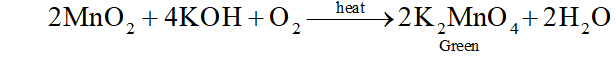The green mass is extracted with water and oxidized to potassium permanganate, electrolytically or by passing chlorine or-ozone into solution. Electrolytic oxidation: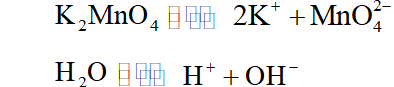During electrolysis manganate ions are oxidized to permanganate at anode.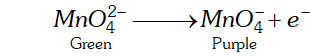At cathode hydrogen gas is liberated$2 \mathrm{H}_{2} \mathrm{O}+2 e^{-} \longrightarrow \mathrm{H}_{2}+2 \mathrm{OH}^{-}$oxidation by chlorine$2 \mathrm{K}_{2} \mathrm{MnO}_{4}+\mathrm{Cl}_{2} \longrightarrow 2 \mathrm{KMnO}_{4}+2 \mathrm{KCl}2 M n O_{4}^{2-}+C l_{2} \longrightarrow 2 M n O_{4}^{2-}+2 C l$or oxidation by ozone$2 \mathrm{K}_{2} \mathrm{MnO}_{4}+\mathrm{O}_{3}+\mathrm{H}_{2} \mathrm{O} \longrightarrow 2 \mathrm{KMn} \mathrm{A}+2 \mathrm{KOH}+\mathrm{O}_{2}2 M n O_{4}^{2-}+O_{3}+H_{2} O \longrightarrow 2 M n O_{4}^{2-}+2 O H^{-}+O_{2}$As a result of oxidation, the green colour of solution changes into purple. The purple solution containing$K M n O_{4}$is concentrated by evaporation which on cooling gives crystals of$\mathrm{KMnO}_{4}$. Q. Write down the number of$3-d$electrons in each of the following ions$: T i^{2+}, V^{2+}, C r^{3+}, M n^{2+} \quad F e^{2+}, F e^{3+}, C o^{2+}, N i^{2+}$and$C u^{2+} .$Indicate how would you expect the five$3 d$orbitals to be occupied for these hydrated ions (octahedral). Ans. (i)$\quad T i^{2+}:[A r] 3 d^{2}$Under the influence of an octahedral ligand field the$d$-orbitals split into two groups of different energies. These are$t_{2 g}$orbitals$(d x y, d x z \text { and } d y z)$and$e_{g}$orbitals$\left(d z^{2} \text { and } d x^{2}-y^{2}\right),$orbitals are of lower energies than orbitals. Thus, the two$d$-electrons in would be present in two of the three orbitals. Thus there would be two unpaired electrons. (ii) The three$d$-electrons in would be present in three different orbitals. Thus, there would be three unpaired electrons. (iii)$-$Three unpaired electrons (iv) - Five unpaired electrons (v)$\quad$- Four unpaired electrons (vi) - Five unpaired electrons (vii)$-\$ Three unpaired electrons (viii) - Two unpaired electrons (ix) - One unpaired electron